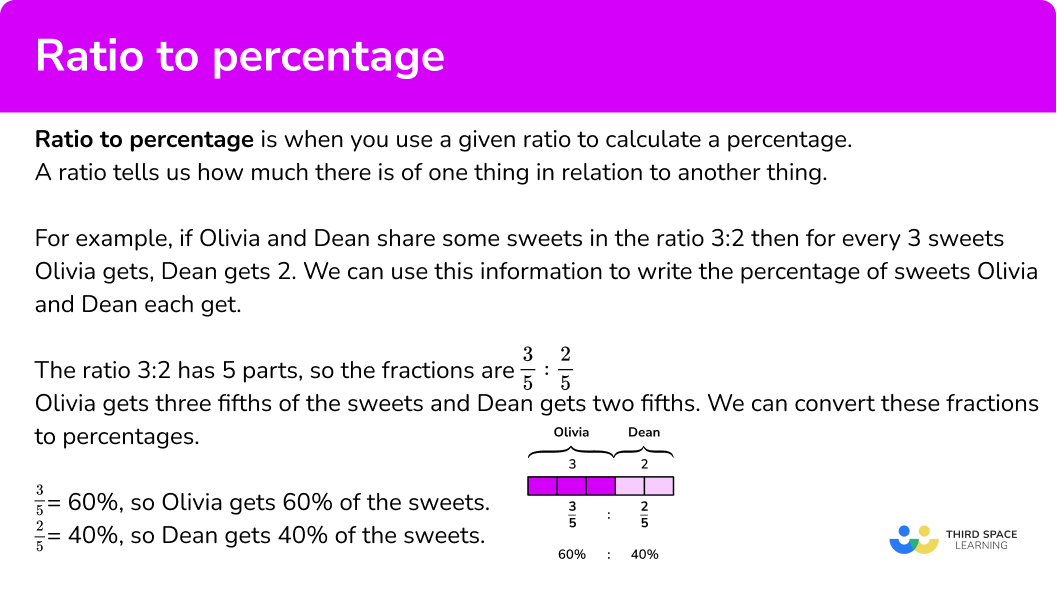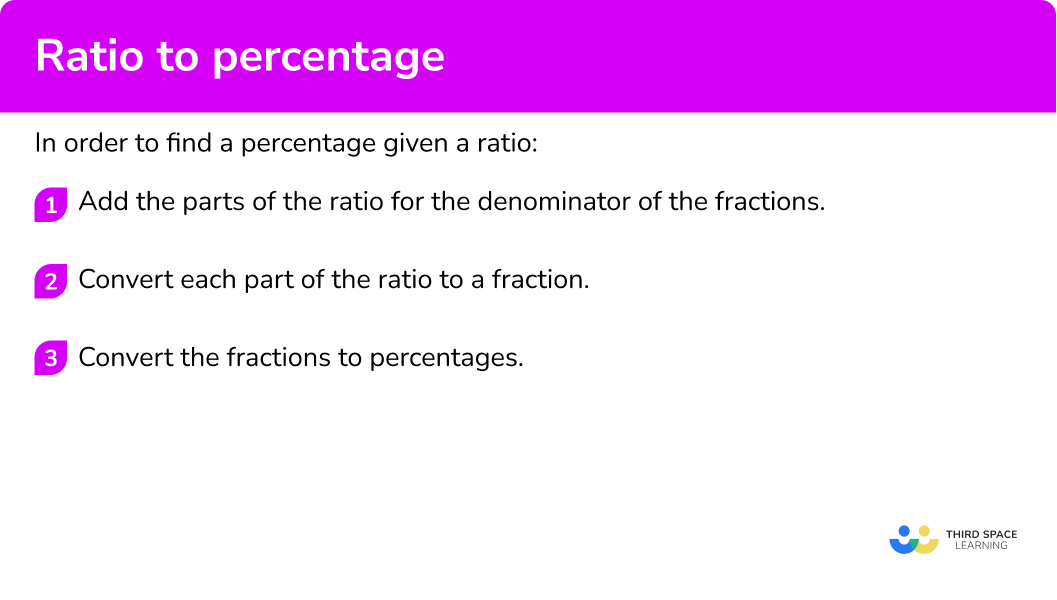GCSE Maths Ratio and Proportion Ratio

Ratio To Percentage

# Ratio To Percentage

Here we will learn about ratio to percentage, including how to convert from ratios to percentages and how to solve word problems involving converting ratios to percentages.

There are also ratio to percentage worksheets based on Edexcel, AQA and OCR exam questions, along with further guidance on where to go next if you’re still stuck.

## What is ratio to percentage?

Ratio to percentage is when you use a given ratio to calculate a percentage.

A ratio tells us how much there is of one thing in relation to another thing.

For example, if Olivia and Dean share some sweets in the ratio 3:2 then for every 3 sweets Olivia gets, Dean gets 2. You can use this information to write the percentage of sweets Olivia and Dean each get.

To find the percentage of sweets they each get, first convert the ratio into fractions.

Step-by-step guide: Ratio to fractions

The ratio 3:2 has 5 parts, so the fractions are \frac{3}{5} : \frac{2}{5}.

Olivia gets three fifths of the sweets and Dean gets two fifths. You can convert these fractions to percentages.

You may be able to recognise what the fractions are as percentages or you may need to use long division to help convert your fractions.

Step-by-step guide: Fractions to percentages

\frac{3}{5}=60\%, so Olivia gets 60\% of the sweets.

\frac{2}{5}=40\%, so Dean gets 40\% of the sweets.

### What is ratio to percentage?## How to convert a ratio to a percentage

In order to convert a ratio to a percentage:

1. Add the parts of the ratio for the denominator of the fractions.
2. Convert each part of the ratio to a fraction.
3. Convert the fractions to percentages.

### Explain how to convert a ratio to a percentage## Related lessons on ratio

Ratio to percentage is part of our series of lessons to support revision on ratio. You may find it helpful to start with the main ratio lesson for a summary of what to expect, or use the step by step guides below for further detail on individual topics. Other lessons in this series include:

## Ratio to percentage examples

### Example 1: converting a two part ratio to percentages

The ratio of blue counters to red counters is 3:1. Write the ratio as percentages.

1. Add the parts of the ratio for the denominator of the fractions.

3+1=4. There are 4 parts in total. The denominator is 4.

2Convert each part of the ratio to a fraction.

3:1 becomes \frac{3}{4}:\frac{1}{4} .

3Convert the fractions to percentages.

\frac{3}{4} and \frac{1}{4} are common fractions for which you need to know the percentage conversions.

\frac{3}{4}:\frac{1}{4}=75\%:25\%

### Example 2: converting a two part ratio to percentages

The ratio of green counters to yellow counters is 3:5. What percentage of the counters are green?

3+5=8. There are 8 parts in total. The denominator is 8.

3:5 becomes \frac{3}{8}:\frac{5}{8}.

0.375=37.5\% and 0.625=62.5\% therefore,

\frac{3}{8}:\frac{5}{8}=37.5\%:62.5\% .

Order is important in ratios and in this ratio the number of green counters is first.

Therefore the percentage of counters that are green is 37.5\%.

### Example 3: converting a three part ratio to percentages

A garden centre sells three types of bulbs; daffodil, tulip and lily, in the ratio 6:9:5. What percentage of the bulbs sold are tulips?

6 + 9 + 5 = 20. There are 20 parts in total. The denominator is 20.

6:9:5 becomes \frac{6}{20}:\frac{9}{20}:\frac{5}{20}.

Writing each fraction with a denominator of 100,

\frac{6}{20}:\frac{9}{20}:\frac{5}{20}=\frac{30}{100}:\frac{45}{100}:\frac{25}{100} .

\frac{6}{20}:\frac{9}{20}:\frac{5}{20}=30\%:45\%:25\%

In this question we are interested in the number of tulips sold, which is the middle value. The percentage that are tulips is 45\%.

### Example 4: solving a problem involving ratio to percentages

In April 2022, Ben and Jacob shared some money in the ratio of their ages. Ben was born in June 2014 and Jacob in January 2019. What percentage of the money does Ben receive?

In April 2022 Ben is 7 and Jacob is 3. The ratio of their ages is 7:3.

7+3=10. There are 10 parts in total. The denominator is 10.

7:3 becomes \frac{7}{10}:\frac{3}{10}.

\frac{7}{10} :\frac{3}{10} = 70\%:30\%

Ben receives 70\% of the money.

### Example 5: solving a problem involving ratio to percentages

The ratio of adults to children in a park is 6:4.

One third of the adults are men. What percentage of the people in the park are women?

6 + 4 = 10. There are 10 parts in total. The denominator is 10.

6:4 becomes \frac{6}{10}:\frac{4}{10}.

\frac{6}{10} : \frac{4}{10} = 60\%:40\%

You have worked out that 60\% of the people are adults.

One third of the adults are men.

\frac{1}{3} of 60\% = 20\%

20\% of the people in the park are men and therefore 60-20=40\% of the people are women.

### Common misconceptions

• Ratios and fractions confusion

For example, the ratio 2:3 is expressed as the fraction \frac{2}{3} and not \frac{2}{5}.

This is a misunderstanding of the sum of the parts of the ratio. Be careful with what the question is asking as the denominator may be the part, or the whole amount.

• Ratio written in the wrong order

Order is important in ratio questions and you must maintain the original order throughout your working and answer.

### Practice ratio to percentage questions

1. Convert the ratio 1:4 to percentages.

80\%:20\%10\%:40\%20\%:80\%40\%:10\%This ratio has 5 parts in total therefore

1:4 = \frac{1}{5}:\frac{4}{5}.

Converting to percentages,

\frac{1}{5}:\frac{4}{5}=20\%:80\%.

2. The ratio of people who own a dog to people who don’t own a dog is 9:11. What percentage of people own a dog?

9\%90\%55\%45\%This ratio has 20 parts therefore

9:11=\frac{9}{20}:\frac{11}{20}.

Converting to percentages,

\frac{9}{20} : \frac{11}{20} = 45\%:55\%.

45\% of people own a dog.

3. The ratio of teachers to students on a school trip is 1:7. What percentage of those on the trip are students?

87.5\%70\%10\%12.5\%The total number of parts in this ratio is 8 therefore

1:7 = \frac{1}{8}:\frac{7}{8}.

Converting to percentages,

\frac{1}{8}:\frac{7}{8} = 12.5\%:87.5\%.

87.5\% of those on the trip are students.

4. Sam, Katrina and Alex share some sweets in the ratio 11:8:6. What percentage of the sweets does Sam get?

24\%55\%11\%44\%The total number of parts in this ratio is 25 therefore

11:8:6 = \frac{11}{25}:\frac{8}{25}:\frac{6}{25}.

Converting to percentages,

\frac{11}{25}:\frac{8}{25}:\frac{6}{25}=44\%:32\%:24\%.

Sam gets 44\% of the sweets.

5. The ratio of the number of boys in a class to the number of girls in a class is 7:3. One fifth of the boys wear glasses. What percentage of the class are boys that wear glasses?

70\%14\%30\%20\%The total number of parts in this ratio is 10 therefore

7:3 = \frac{7}{10} : \frac{3}{10}.

Converting to percentages,

\frac{7}{10} : \frac{3}{10} = 70\%:30\%.

70\% of the class are boys and one fifth of the boys wear glasses.

\frac{1}{5} of 70\% = 14\% .

14\% of the class are boys who wear glasses.

6. Tony and Anne share some money in the ratio 23:27.

Anne gives a quarter of her share to her friend James.

What percentage of the money does James get?

5.75\%11.5\%23\%46\%The total number of parts in this ratio is 50 therefore

23:27 = \frac{23}{50} : \frac{27}{50}.

Converting to percentages,

\frac{23}{50} : \frac{27}{50} = 46\%:54\%.

Anne gets 46\% of the money and gives one quarter of her share to James.

\frac{1}{4} of 46\% = 11.5\% .

James gets 11.5\% of the money.

### Ratio to percentage GCSE questions

1. Write the following ratio as percentages.

13:7

(2 marks)

\frac{13}{20} : \frac{7}{20}

(1)

65\%:35\%

(1)

2. William and Matthew share some money in the ratio 3:7. Matthew gives two fifths of his money to Ellie.

Who receives the most money? Show how you decide.

(4 marks)

William 30\%, Matthew 70\%

(1)

\frac{2}{5} of 70\%=28\% .

(1)

Matthew 42\%, Ellie 28\%

(1)

(1)

3. The ratio of men to women working for an accountancy firm is 5:3.

One fifth of the women are managers and 10\% of the men are managers.

What percentage of all employees are managers?

(4 marks)

For converting 5:3 to fractions or percentages, for example 62.5\%:37.5\%.

(1)

10\% of 62.5\%=6.25\%

(1)

\frac{1}{5} of 37.5\%=7.5\%

(1)

6.25\%+7.5\%=13.75\%

(1)

## Learning checklist

You have now learned how to:

• Convert ratios to percentages and solve problems involving ratios and percentages

## Still stuck?

Prepare your KS4 students for maths GCSEs success with Third Space Learning. Weekly online one to one GCSE maths revision lessons delivered by expert maths tutors.

Find out more about our GCSE maths tuition programme.